# Excel 2007 Excel Basics Excel spreadsheets organize information

• Slides: 47Excel 2007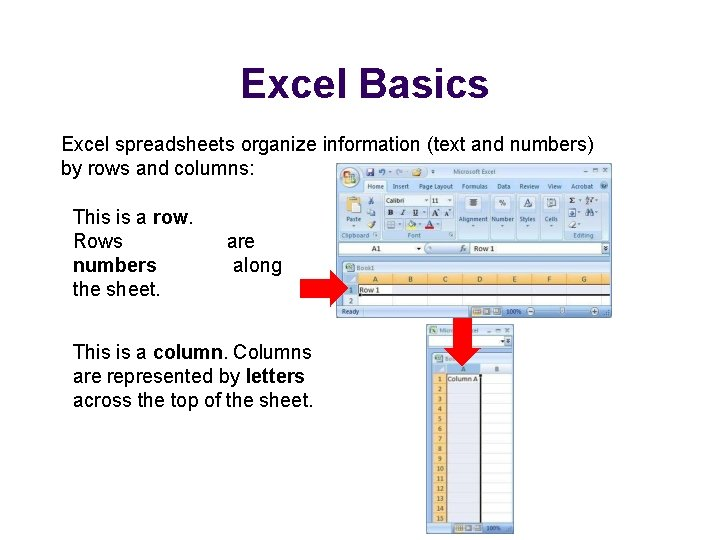Excel Basics Excel spreadsheets organize information (text and numbers) by rows and columns: This is a row. Rows numbers the sheet. are along This is a column. Columns are represented by letters across the top of the sheet. represented the side by ofExcel Basics A cell is the intersection between a column and a row. Each cell is named for the column letter and row number that intersect to make it.Data Entry There are two ways to enter information into a cell: 1. Type directly into the cell. Click on a cell, and type in the data (numbers or text) and press Enter. 2. Type into the formula bar. Click on a cell, and then click in the formula bar (the space next to the ). Now type the data into the bar and press Enter.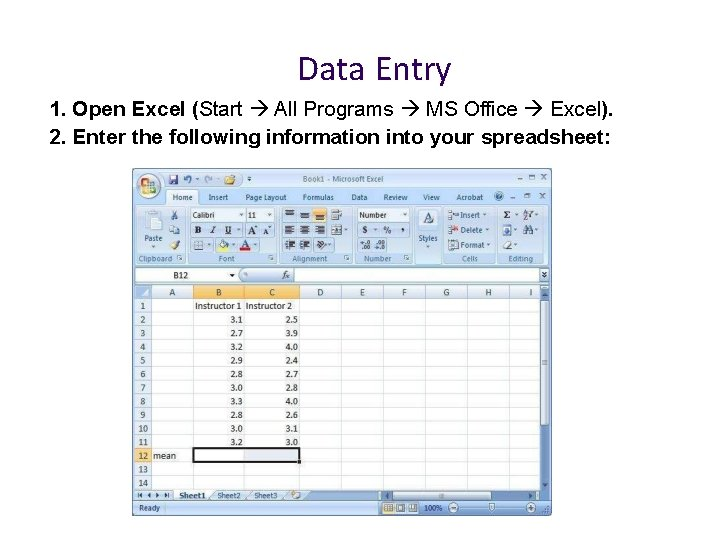Data Entry 1. Open Excel (Start All Programs MS Office Excel). 2. Enter the following information into your spreadsheet:Formulas and Functions Formulas are equations that perform calculations in your spreadsheet. Formulas always begin with an equals sign (=). When you enter an equals sign into a cell, you are basically telling Excel to “calculate this. ” Functions are Excel-defined formulas. They take data you select and enter, perform calculations on them, and return value(s).More on Functions All functions have a common format – the equals sign followed by the function name followed by the input in parentheses. The input for a function can be either: A set of numbers (e. g. , “=AVERAGE(2, 3, 4, 5)”) This tells Excel to calculate the average of these numbers. A reference to cell(s) (e. g. , “=AVERAGE(B 1: B 18) or “=AVERAGE (B 1, B 2, B 3, B 4, B 5, B 6, B 7, B 8)” This tells Excel to calculate the average of the data that appear in all the cells from B 1 to B 8. You can either type these cell references in by hand or by clicking and dragging with your mouse to select the cells.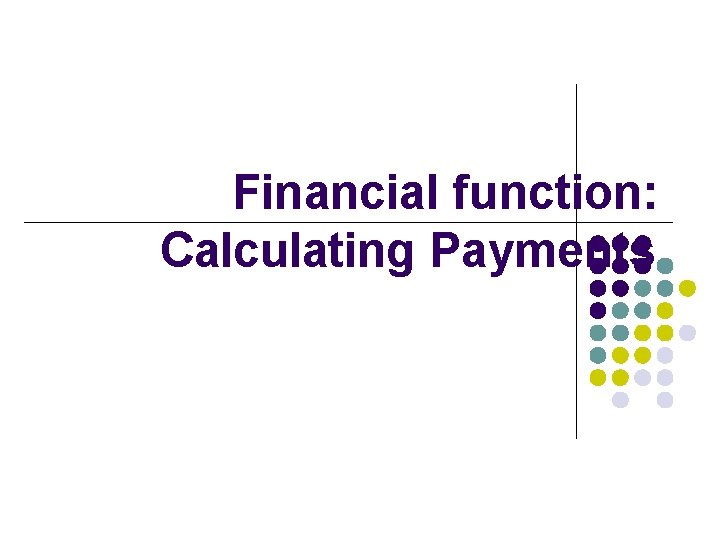Financial function: Calculating PaymentsPMT The PMT function returns a payment amount, so you can use it to calculate the monthly payment due on a personal loan. PMT Syntax The PMT function has the following syntax: PMT(rate, nper, pv, [fv], [type]) Rate is the interest rate for the loan. Nper is the total number of payments for the loan. Pv is the present value; also known as the principal. Fv is optional. It is the future value, or the balance that you want to have left after the last payment. If fv is omitted, the fv is assumed to be zero. Type is optional. If omitted, it is assumed to be zero, and payments are due at the end of the period. Use 1 in this argument if payments are due at the beginning of the period.=PMT(rate, nper, pv, fv, type) The interest rate is shown as 6%, but remember that you don’t pay it all at once – you only pay 1/12 th of it at a time, so when you put it in the formula, you do it like this: . 06/12 that means 6 percent divided by 12=PMT(rate, nper, pv, fv, type) How much you owe at the end of the loan? =PMT(. 06/12, 36, 30000, 0, 0) Payment made at the end of the month 6% interest 36 months You’re paying \$30000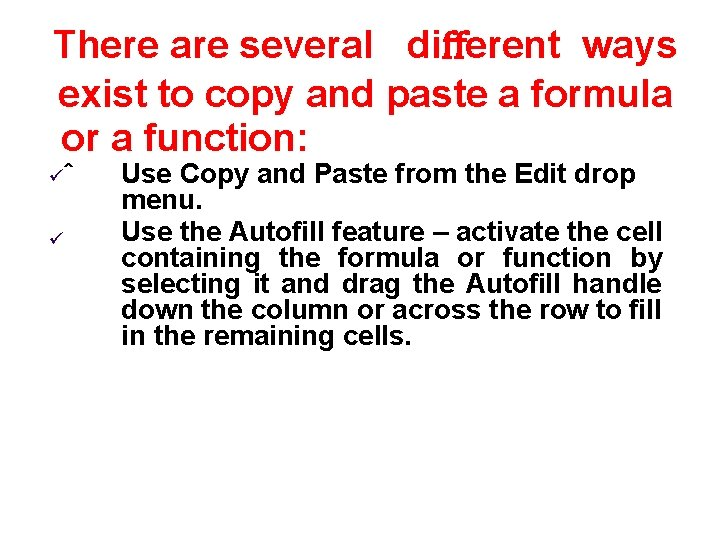There are several diﬀerent ways exist to copy and paste a formula or a function: ˆ Use Copy and Paste from the Edit drop menu. Use the Autoﬁll feature – activate the cell containing the formula or function by selecting it and drag the Autoﬁll handle down the column or across the row to ﬁll in the remaining cells.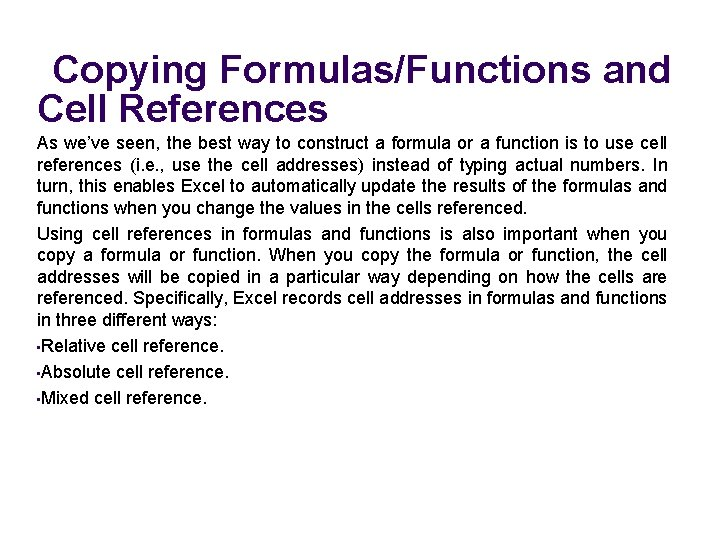Copying Formulas/Functions and Cell References As we’ve seen, the best way to construct a formula or a function is to use cell references (i. e. , use the cell addresses) instead of typing actual numbers. In turn, this enables Excel to automatically update the results of the formulas and functions when you change the values in the cells referenced. Using cell references in formulas and functions is also important when you copy a formula or function. When you copy the formula or function, the cell addresses will be copied in a particular way depending on how the cells are referenced. Speciﬁcally, Excel records cell addresses in formulas and functions in three different ways: • Relative cell reference. • Absolute cell reference. • Mixed cell reference.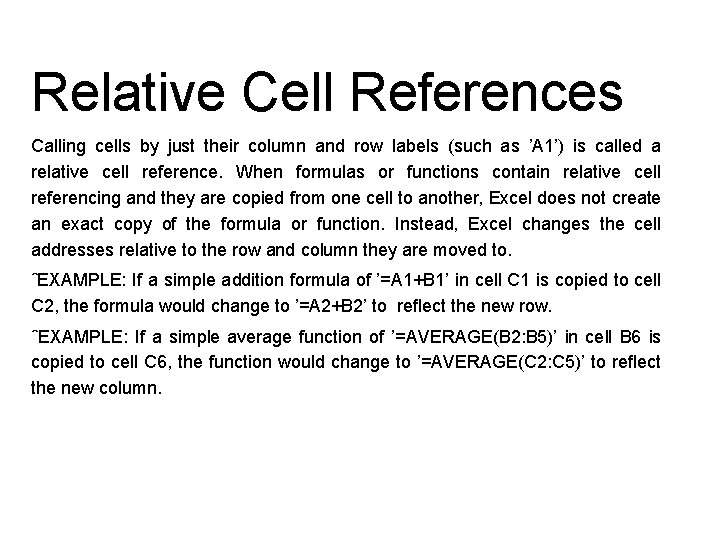Relative Cell References Calling cells by just their column and row labels (such as ’A 1’) is called a relative cell reference. When formulas or functions contain relative cell referencing and they are copied from one cell to another, Excel does not create an exact copy of the formula or function. Instead, Excel changes the cell addresses relative to the row and column they are moved to. ˆEXAMPLE: If a simple addition formula of ’=A 1+B 1’ in cell C 1 is copied to cell C 2, the formula would change to ’=A 2+B 2’ to reﬂect the new row. ˆEXAMPLE: If a simple average function of ’=AVERAGE(B 2: B 5)’ in cell B 6 is copied to cell C 6, the function would change to ’=AVERAGE(C 2: C 5)’ to reﬂect the new column.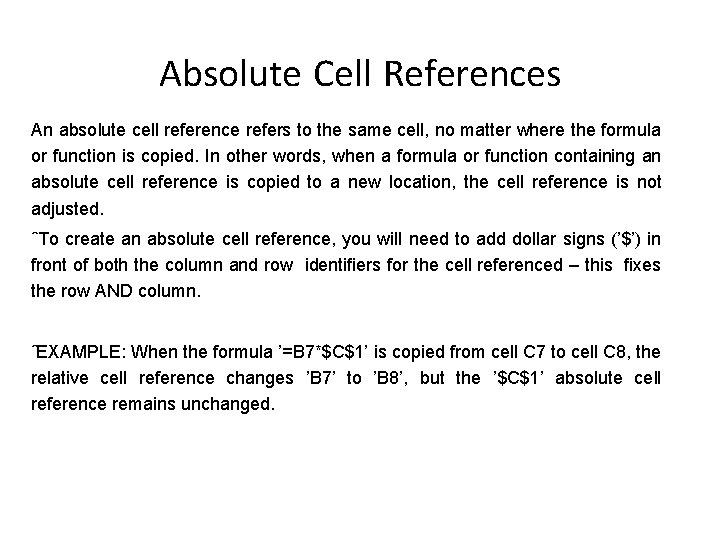Absolute Cell References An absolute cell reference refers to the same cell, no matter where the formula or function is copied. In other words, when a formula or function containing an absolute cell reference is copied to a new location, the cell reference is not adjusted. ˆTo create an absolute cell reference, you will need to add dollar signs (’\$’) in front of both the column and row identiﬁers for the cell referenced – this ﬁxes the row AND column. ˆEXAMPLE: When the formula ’=B 7*\$C\$1’ is copied from cell C 7 to cell C 8, the relative cell reference changes ’B 7’ to ’B 8’, but the ’\$C\$1’ absolute cell reference remains unchanged.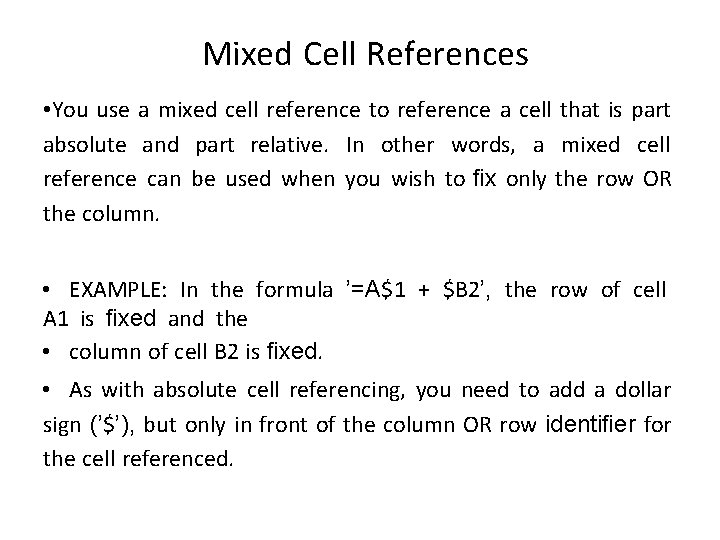Mixed Cell References • You use a mixed cell reference to reference a cell that is part absolute and part relative. In other words, a mixed cell reference can be used when you wish to ﬁx only the row OR the column. • EXAMPLE: In the formula ’=A\$1 + \$B 2’, the row of cell A 1 is ﬁxed and the • column of cell B 2 is ﬁxed. • As with absolute cell referencing, you need to add a dollar sign (’\$’), but only in front of the column OR row identiﬁer for the cell referenced.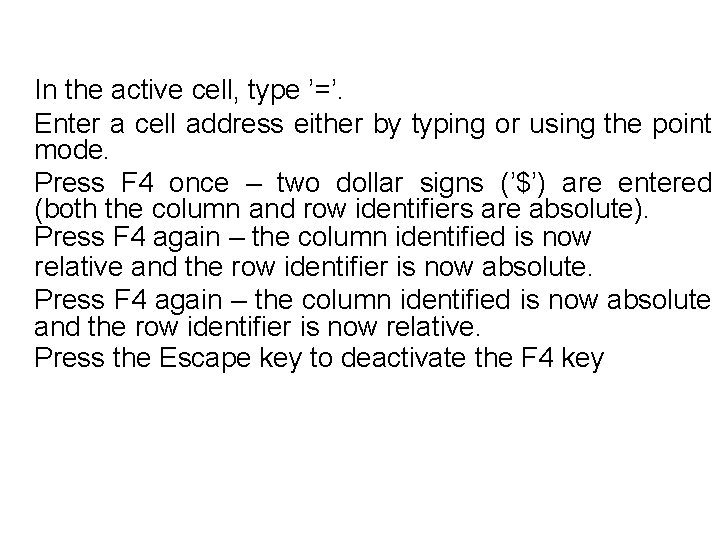In the active cell, type ’=’. Enter a cell address either by typing or using the point mode. Press F 4 once – two dollar signs (’\$’) are entered (both the column and row identiﬁers are absolute). Press F 4 again – the column identiﬁed is now relative and the row identiﬁer is now absolute. Press F 4 again – the column identiﬁed is now absolute and the row identiﬁer is now relative. Press the Escape key to deactivate the F 4 key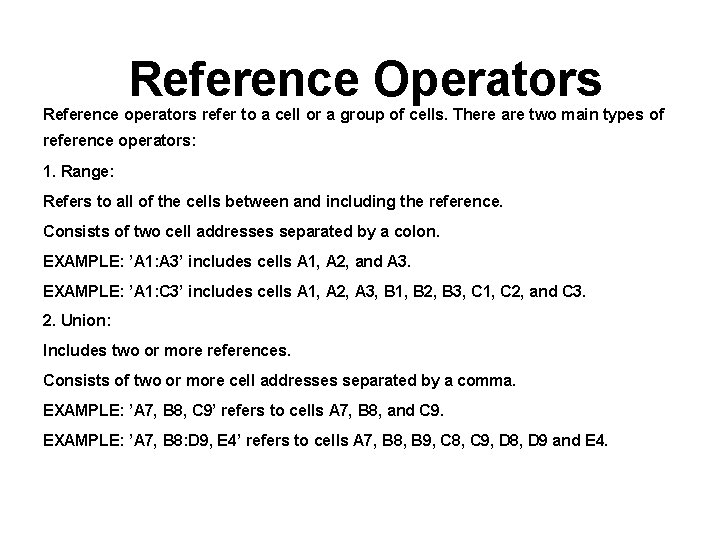Reference Operators Reference operators refer to a cell or a group of cells. There are two main types of reference operators: 1. Range: Refers to all of the cells between and including the reference. Consists of two cell addresses separated by a colon. EXAMPLE: ’A 1: A 3’ includes cells A 1, A 2, and A 3. EXAMPLE: ’A 1: C 3’ includes cells A 1, A 2, A 3, B 1, B 2, B 3, C 1, C 2, and C 3. 2. Union: Includes two or more references. Consists of two or more cell addresses separated by a comma. EXAMPLE: ’A 7, B 8, C 9’ refers to cells A 7, B 8, and C 9. EXAMPLE: ’A 7, B 8: D 9, E 4’ refers to cells A 7, B 8, B 9, C 8, C 9, D 8, D 9 and E 4.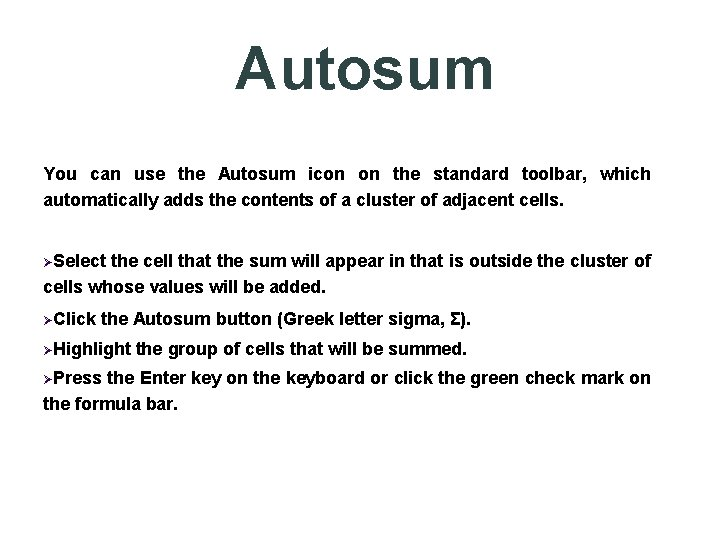Autosum You can use the Autosum icon on the standard toolbar, which automatically adds the contents of a cluster of adjacent cells. Select the cell that the sum will appear in that is outside the cluster of cells whose values will be added. Click the Autosum button (Greek letter sigma, Σ). Highlight Press the group of cells that will be summed. the Enter key on the keyboard or click the green check mark on the formula bar.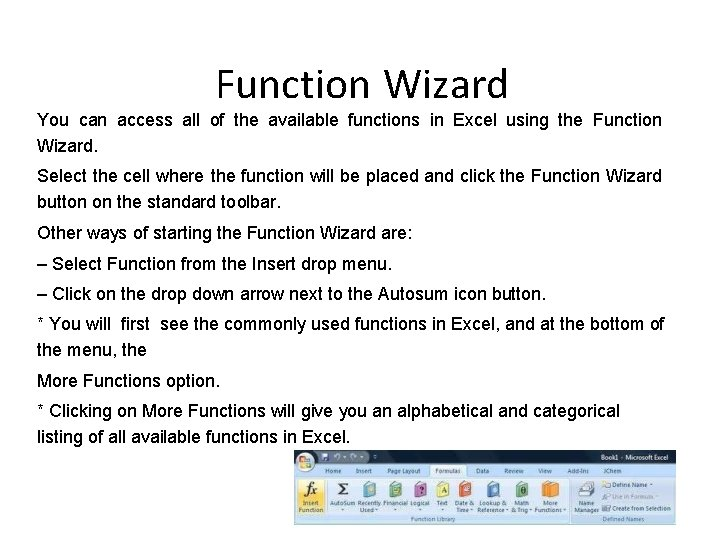Function Wizard You can access all of the available functions in Excel using the Function Wizard. Select the cell where the function will be placed and click the Function Wizard button on the standard toolbar. Other ways of starting the Function Wizard are: – Select Function from the Insert drop menu. – Click on the drop down arrow next to the Autosum icon button. * You will ﬁrst see the commonly used functions in Excel, and at the bottom of the menu, the More Functions option. * Clicking on More Functions will give you an alphabetical and categorical listing of all available functions in Excel.Linking Worksheets You may want to use the value from a cell in another worksheet within the same workbook in a formula. � Use the format ’sheetname!celladdress’. ˆEXAMPLE: The value of cell A 1 in the current worksheet and cell A 2 in the second worksheet can be added using the formula ’=A 1+Sheet 2!A 2’.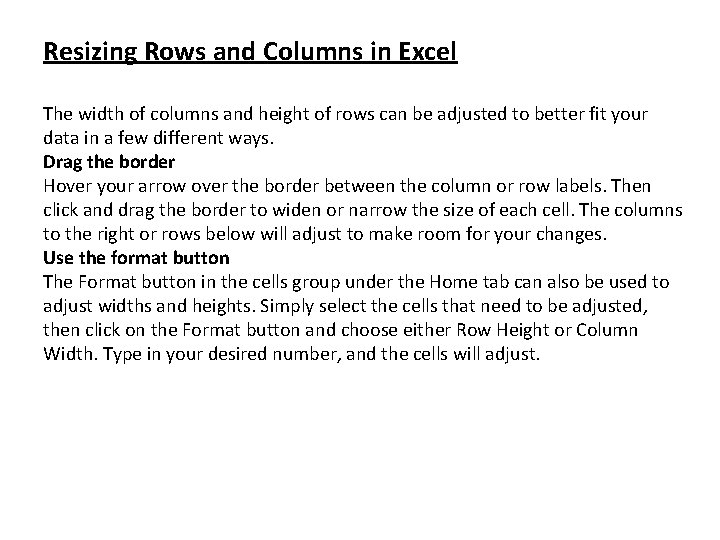Resizing Rows and Columns in Excel The width of columns and height of rows can be adjusted to better fit your data in a few different ways. Drag the border Hover your arrow over the border between the column or row labels. Then click and drag the border to widen or narrow the size of each cell. The columns to the right or rows below will adjust to make room for your changes. Use the format button The Format button in the cells group under the Home tab can also be used to adjust widths and heights. Simply select the cells that need to be adjusted, then click on the Format button and choose either Row Height or Column Width. Type in your desired number, and the cells will adjust.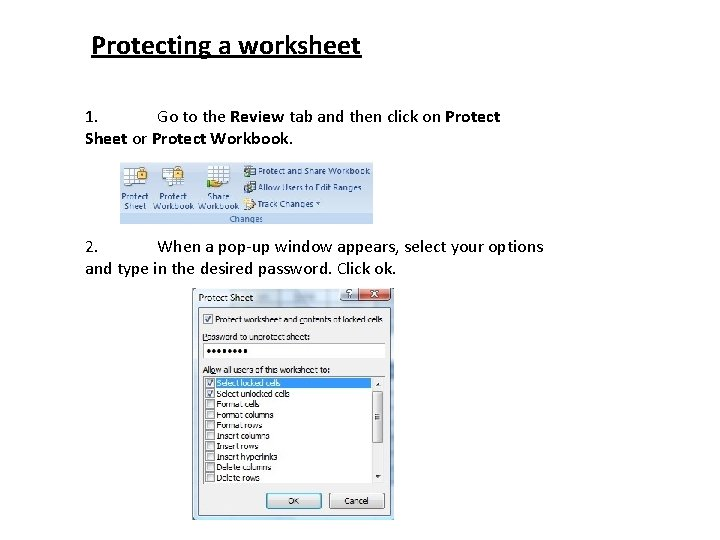Protecting a worksheet 1. Go to the Review tab and then click on Protect Sheet or Protect Workbook. 2. When a pop-up window appears, select your options and type in the desired password. Click ok.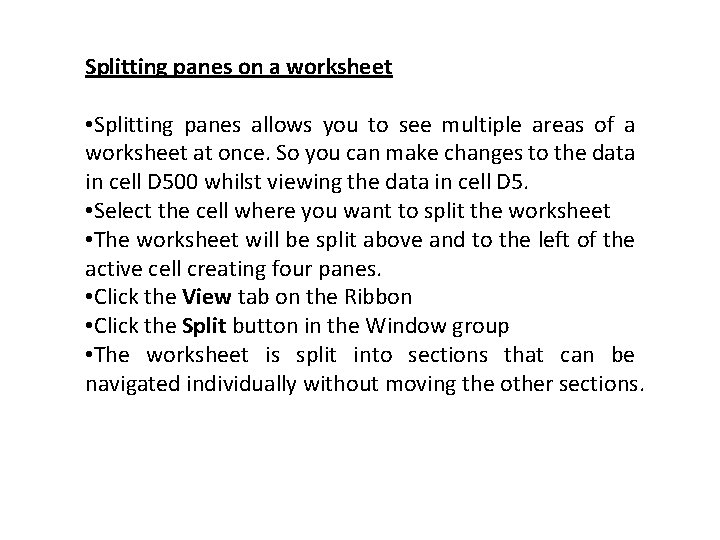Splitting panes on a worksheet • Splitting panes allows you to see multiple areas of a worksheet at once. So you can make changes to the data in cell D 500 whilst viewing the data in cell D 5. • Select the cell where you want to split the worksheet • The worksheet will be split above and to the left of the active cell creating four panes. • Click the View tab on the Ribbon • Click the Split button in the Window group • The worksheet is split into sections that can be navigated individually without moving the other sections.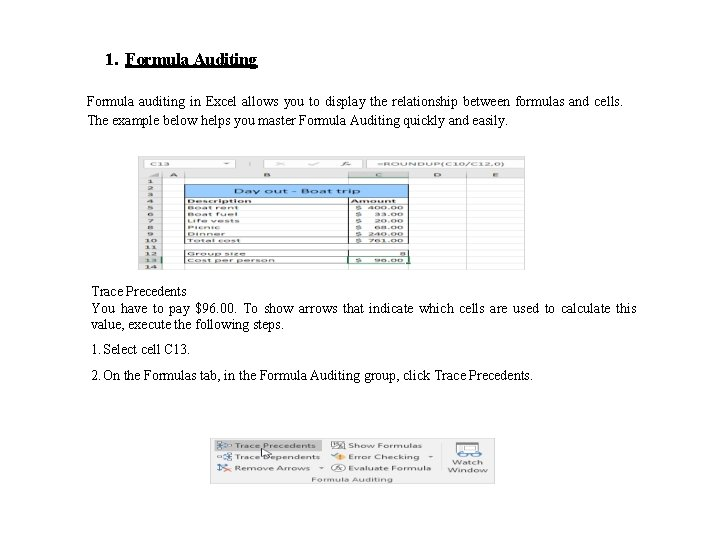1. Formula Auditing Formula auditing in Excel allows you to display the relationship between formulas and cells. The example below helps you master Formula Auditing quickly and easily. Trace Precedents You have to pay \$96. 00. To show arrows that indicate which cells are used to calculate this value, execute the following steps. 1. Select cell C 13. 2. On the Formulas tab, in the Formula Auditing group, click Trace Precedents.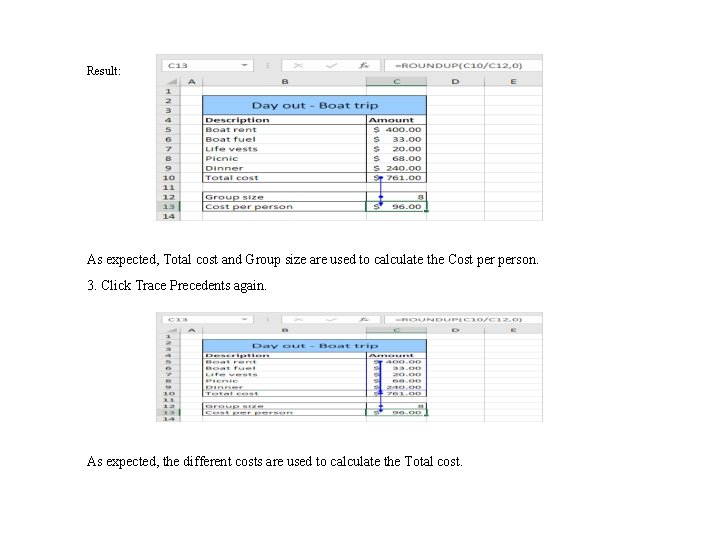Result: As expected, Total cost and Group size are used to calculate the Cost person. 3. Click Trace Precedents again. As expected, the different costs are used to calculate the Total cost.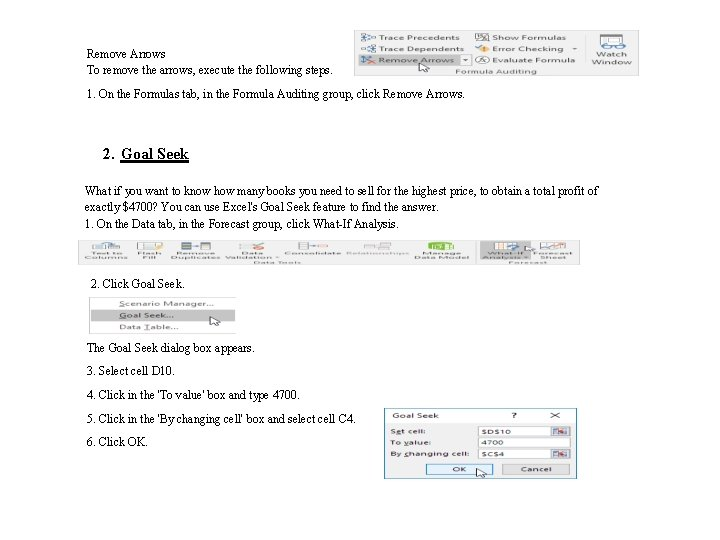Remove Arrows To remove the arrows, execute the following steps. 1. On the Formulas tab, in the Formula Auditing group, click Remove Arrows. 2. Goal Seek What if you want to know how many books you need to sell for the highest price, to obtain a total profit of exactly \$4700? You can use Excel's Goal Seek feature to find the answer. 1. On the Data tab, in the Forecast group, click What-If Analysis. 2. Click Goal Seek. The Goal Seek dialog box appears. 3. Select cell D 10. 4. Click in the 'To value' box and type 4700. 5. Click in the 'By changing cell' box and select cell C 4. 6. Click OK.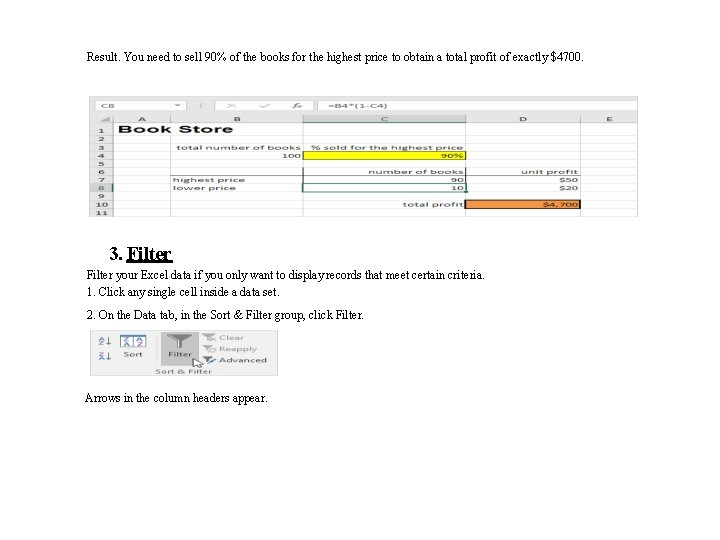Result. You need to sell 90% of the books for the highest price to obtain a total profit of exactly \$4700. 3. Filter your Excel data if you only want to display records that meet certain criteria. 1. Click any single cell inside a data set. 2. On the Data tab, in the Sort & Filter group, click Filter. Arrows in the column headers appear.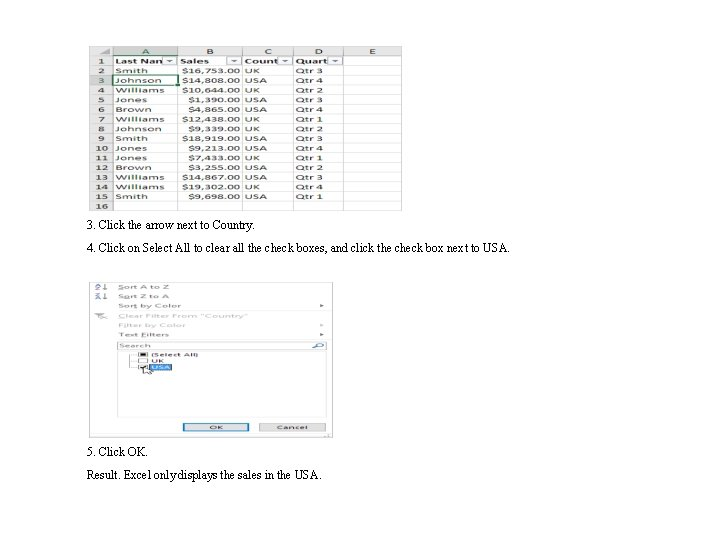3. Click the arrow next to Country. 4. Click on Select All to clear all the check boxes, and click the check box next to USA. 5. Click OK. Result. Excel only displays the sales in the USA.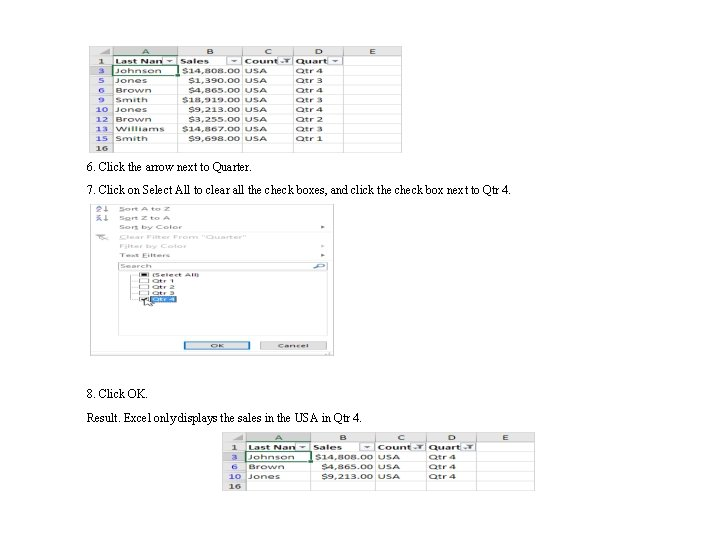6. Click the arrow next to Quarter. 7. Click on Select All to clear all the check boxes, and click the check box next to Qtr 4. 8. Click OK. Result. Excel only displays the sales in the USA in Qtr 4.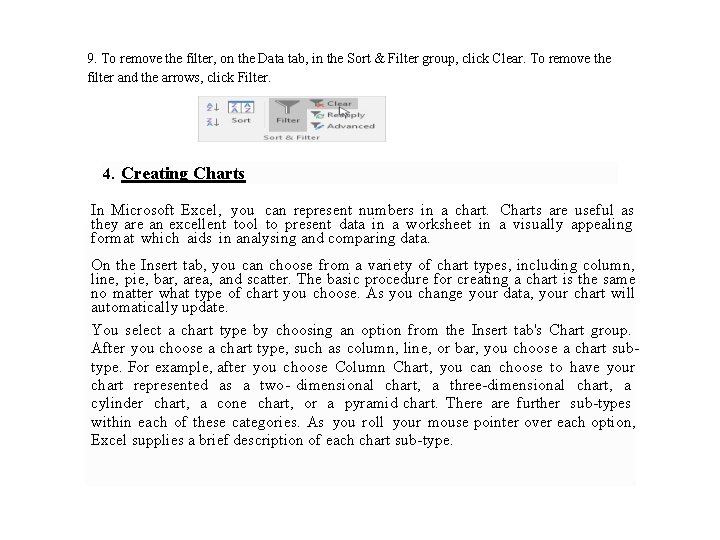9. To remove the filter, on the Data tab, in the Sort & Filter group, click Clear. To remove the filter and the arrows, click Filter. 4. Creating Charts In Microsoft Excel, you can represent numbers in a chart. Charts are useful as they are an excellent tool to present data in a worksheet in a visually appealing format which aids in analysing and comparing data. On the Insert tab, you can choose from a variety of chart types, including column, line, pie, bar, area, and scatter. The basic procedure for creating a chart is the same no matter what type of chart you choose. As you change your data, your chart will automatically update. You select a chart type by choosing an option from the Insert tab's Chart group. After you choose a chart type, such as column, line, or bar, you choose a chart subtype. For example, after you choose Column Chart, you can choose to have your chart represented as a two- dimensional chart, a three-dimensional chart, a cylinder chart, a cone chart, or a pyramid chart. There are further sub-types within each of these categories. As you roll your mouse pointer over each option, Excel supplies a brief description of each chart sub-type.Some chart types available in Excel are: 1. Pie Chart – It shows the proportional size of data that make up a data series and is useful when we want to emphasize a significant element. 2. Column Chart – A column chart shows data changes over a period of time or illustrates comparisons among items. 3. Bar Chart – It illustrates comparisons among individual items. Categories are organized vertically, values horizontally to focus on comparing values and to place less emphasis on time. 4. Line Chart: The Line Chart is especially effective in displaying trends. In a Line Chart, the vertical axis (Y-axis) always displays numeric values and the horizontal axis (X-axis) displays time or other category.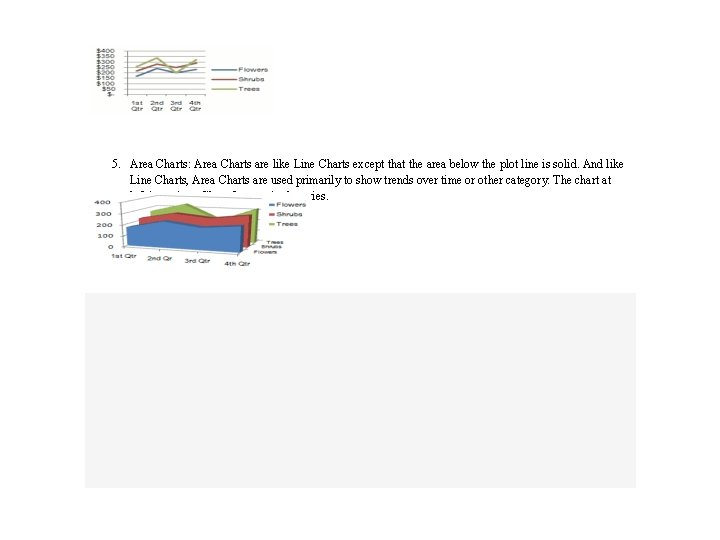5. Area Charts: Area Charts are like Line Charts except that the area below the plot line is solid. And like Line Charts, Area Charts are used primarily to show trends over time or other category. The chart at left is an Area Chart for our single series.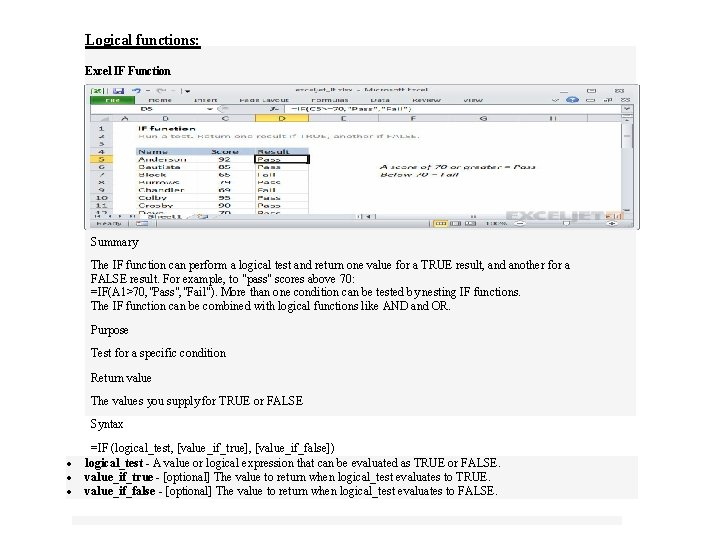Logical functions: Excel IF Function Summary The IF function can perform a logical test and return one value for a TRUE result, and another for a FALSE result. For example, to "pass" scores above 70: =IF(A 1>70, "Pass", "Fail"). More than one condition can be tested by nesting IF functions. The IF function can be combined with logical functions like AND and OR. Purpose Test for a specific condition Return value The values you supply for TRUE or FALSE Syntax =IF (logical_test, [value_if_true], [value_if_false]) logical_test - A value or logical expression that can be evaluated as TRUE or FALSE. Arguments - [optional] The value to return when logical_test evaluates to TRUE. value_if_true value_if_false - [optional] The value to return when logical_test evaluates to FALSE.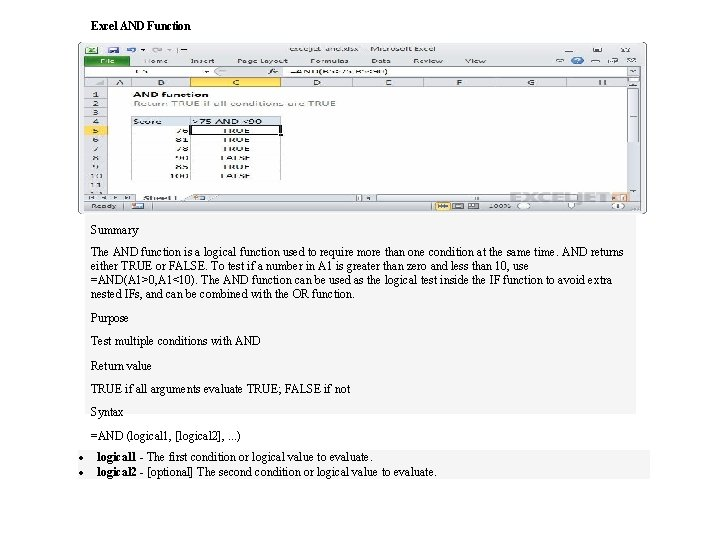Excel AND Function Summary The AND function is a logical function used to require more than one condition at the same time. AND returns either TRUE or FALSE. To test if a number in A 1 is greater than zero and less than 10, use =AND(A 1>0, A 1<10). The AND function can be used as the logical test inside the IF function to avoid extra nested IFs, and can be combined with the OR function. Purpose Test multiple conditions with AND Return value TRUE if all arguments evaluate TRUE; FALSE if not Syntax =AND (logical 1, [logical 2], . . . ) Arguments logical 1 - The first condition or logical value to evaluate. logical 2 - [optional] The secondition or logical value to evaluate.Excel OR Function Summary The OR function is a logical function to test multiple conditions at the same time. OR returns either TRUE or FALSE. For example, to test A 1 for either "x" or "y", use =OR(A 1="x", A 1="y"). The OR function can be used as the logical test inside the IF function to avoid extra nested IFs, and can be combined with the AND function. Purpose Test multiple conditions with OR Return value TRUE if any arguments evaluate TRUE; FALSE if not. Syntax =OR (logical 1, [logical 2], . . . ) Arguments logical 1 - The first condition or logical value to evaluate. logical 2 - [optional] The secondition or logical value to evaluate.Excel NOT Function Summary The Excel NOT function returns the opposite of a given logical or boolean value. When given TRUE, NOT returns FALSE. When given FALSE, NOT returns TRUE. Use the NOT function to reverse a logical value. Purpose Reverse arguments or results Return value A reversed logical value Syntax =NOT (logical) Arguments logical - A value or logical expression that can be evaluated as TRUE or FALSE.Statistical functions: Excel AVERAGE Function Purpose Get the average of a group of numbers Return value A number representing the average. Syntax =AVERAGE (number 1, [number 2], . . . ) Arguments number 1 - A number or cell reference that refers to numeric values. number 2 - [optional] A number or cell reference that refers to numeric values.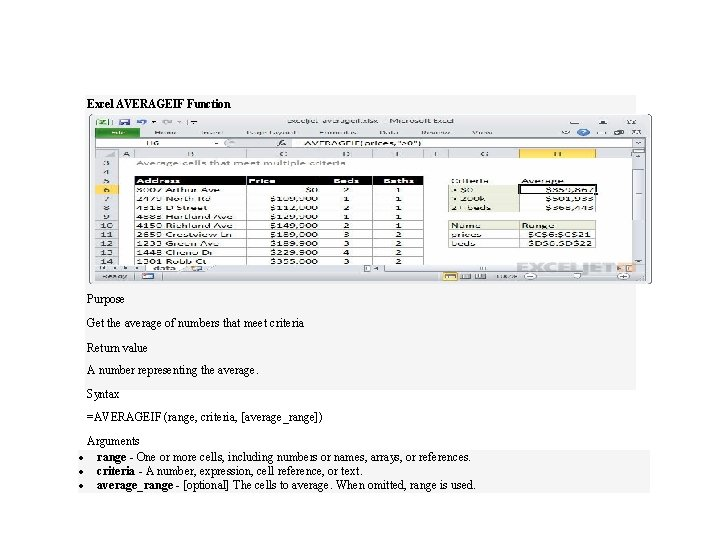Excel AVERAGEIF Function Purpose Get the average of numbers that meet criteria Return value A number representing the average. Syntax =AVERAGEIF (range, criteria, [average_range]) Arguments range - One or more cells, including numbers or names, arrays, or references. criteria - A number, expression, cell reference, or text. average_range - [optional] The cells to average. When omitted, range is used.Excel AVERAGEA Function Purpose Get the average of a group of numbers and text Return value A number representing the average. Syntax =AVERAGEA (value 1, [value 2], . . . ) Arguments value 1 - A value or reference to a value that can be evaluated as a number. value 2 - [optional] A value or reference to a value that can be evaluated as a number.Excel COUNT Function Purpose Count numbers Return value A number representing a count of numbers. Syntax =COUNT (value 1, [value 2], . . . ) Arguments value 1 - An item, cell reference, or range. value 2 - [optional] An item, cell reference, or range.Excel COUNTA Function Purpose Count the number of non-blank cells Return value A number representing non-blank cells. Syntax =COUNTA (value 1, [value 2], . . . ) Arguments value 1 - An item, cell reference, or range. value 2 - [optional] An item, cell reference, or range.Excel COUNTIF Function Summary COUNTIF is a function to count cells that meet a single criteria. COUNTIF can be used to count cells with dates, numbers, and text that match specific criteria. The COUNTIF function supports logical operators (>, <, <>, =) and wildcards (*, ? ) for partial matching. Purpose Count cells that match criteria Return value A number representing cells counted. Syntax =COUNTIF (range, criteria) Arguments range - The range of cells to count. criteria - The criteria that controls which cells should be counted.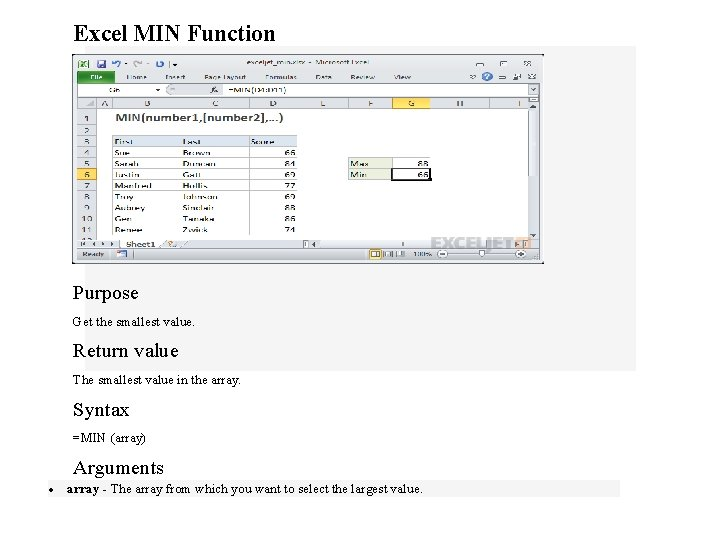Excel MIN Function Purpose Get the smallest value. Return value The smallest value in the array. Syntax =MIN (array) Arguments array - The array from which you want to select the largest value.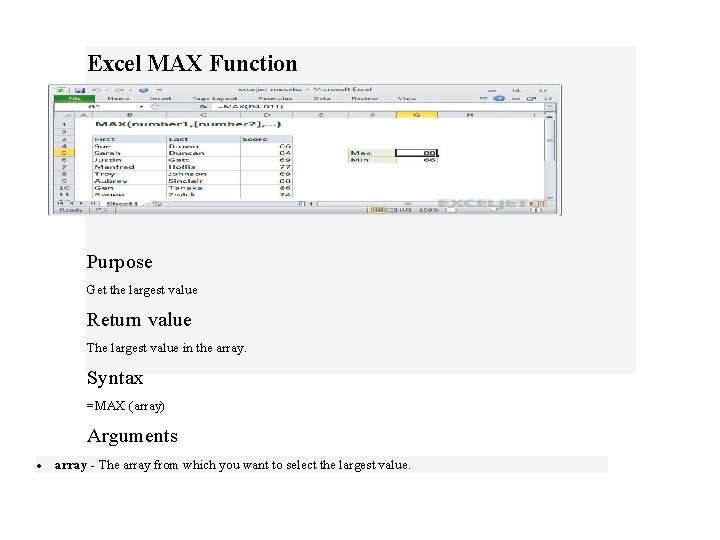Excel MAX Function Purpose Get the largest value Return value The largest value in the array. Syntax =MAX (array) Arguments array - The array from which you want to select the largest value.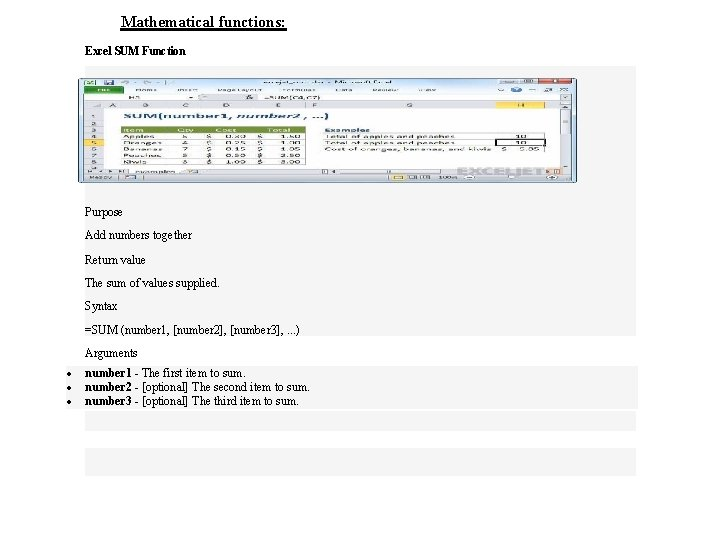Mathematical functions: Excel SUM Function Purpose Add numbers together Return value The sum of values supplied. Syntax =SUM (number 1, [number 2], [number 3], . . . ) Arguments number 1 - The first item to sum. number 2 - [optional] The second item to sum. number 3 - [optional] The third item to sum.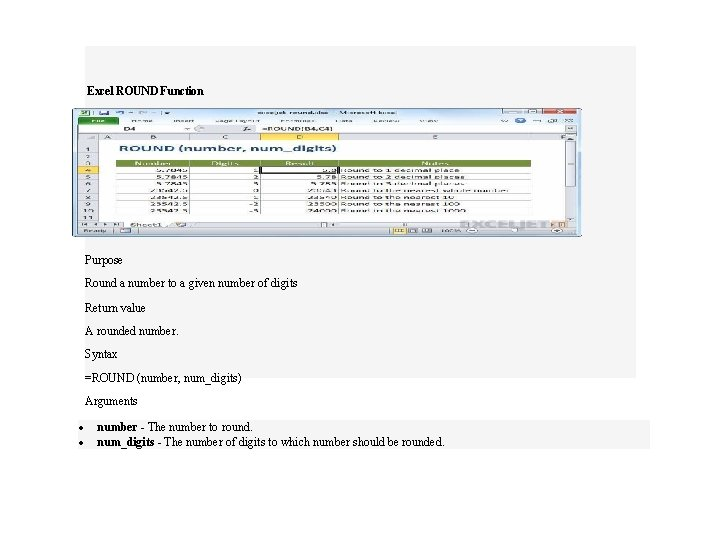Excel ROUND Function Purpose Round a number to a given number of digits Return value A rounded number. Syntax =ROUND (number, num_digits) Arguments number - The number to round. num_digits - The number of digits to which number should be rounded.